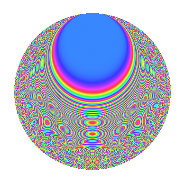# Properties

 Label 8027.2.a.fLevel 8027 Weight 2 Character orbit 8027.a Self dual Yes Analytic conductor 64.096 Analytic rank 0 Dimension 176 CM No

# Related objects

## Newspace parameters

 Level: $$N$$ = $$8027 = 23 \cdot 349$$ Weight: $$k$$ = $$2$$ Character orbit: $$[\chi]$$ = 8027.a (trivial)

## Newform invariants

 Self dual: Yes Analytic conductor: $$64.0959177025$$ Analytic rank: $$0$$ Dimension: $$176$$ Fricke sign: $$-1$$ Sato-Tate group: $\mathrm{SU}(2)$

## $q$-expansion

The dimension is sufficiently large that we do not compute an algebraic $$q$$-expansion, but we have computed the trace expansion.

 $$\operatorname{Tr}(f)(q) =$$ $$176q + 19q^{2} + 22q^{3} + 203q^{4} + 28q^{5} + 9q^{6} + 30q^{7} + 51q^{8} + 204q^{9} + O(q^{10})$$ $$\operatorname{Tr}(f)(q) =$$ $$176q + 19q^{2} + 22q^{3} + 203q^{4} + 28q^{5} + 9q^{6} + 30q^{7} + 51q^{8} + 204q^{9} + 18q^{10} + 11q^{11} + 46q^{12} + 87q^{13} - 6q^{14} + 29q^{15} + 257q^{16} + 14q^{17} + 72q^{18} + 34q^{19} + 45q^{20} + 23q^{21} + 62q^{22} + 176q^{23} + 33q^{24} + 272q^{25} + 31q^{26} + 82q^{27} + 80q^{28} + 75q^{29} - 30q^{30} + 73q^{31} + 71q^{32} + 30q^{33} + 23q^{34} + 44q^{35} + 264q^{36} + 236q^{37} - 21q^{38} + 17q^{39} + 43q^{40} + 51q^{41} + 38q^{42} + 51q^{43} + 12q^{44} + 127q^{45} + 19q^{46} + 45q^{47} + 61q^{48} + 268q^{49} + 55q^{50} - 3q^{51} + 166q^{52} + 63q^{53} - 32q^{54} + 11q^{55} - 9q^{56} + 72q^{57} + 98q^{58} + 95q^{59} - 7q^{60} + 73q^{61} + 12q^{62} + 19q^{63} + 365q^{64} + 19q^{65} - 28q^{66} + 138q^{67} + 16q^{68} + 22q^{69} + 100q^{70} + 85q^{71} + 129q^{72} + 118q^{73} - 21q^{74} - 12q^{75} + 52q^{76} + 75q^{77} + 97q^{78} + 74q^{79} + 8q^{80} + 280q^{81} + 67q^{82} + 10q^{83} - 51q^{84} + 169q^{85} - 39q^{86} - 6q^{87} + 159q^{88} + 38q^{89} + 22q^{90} + 90q^{91} + 203q^{92} + 230q^{93} + 63q^{94} + 30q^{95} + 107q^{96} + 161q^{97} + 58q^{98} + 7q^{99} + O(q^{100})$$

## Embeddings

For each embedding $$\iota_m$$ of the coefficient field, the values $$\iota_m(a_n)$$ are shown below.

For more information on an embedded modular form you can click on its label.

Label $$a_{2}$$ $$a_{3}$$ $$a_{4}$$ $$a_{5}$$ $$a_{6}$$ $$a_{7}$$ $$a_{8}$$ $$a_{9}$$ $$a_{10}$$
1.1 −2.81854 −0.267983 5.94415 −3.79404 0.755319 −0.324133 −11.1167 −2.92819 10.6936
1.2 −2.77938 1.07296 5.72495 0.733926 −2.98217 −2.15808 −10.3531 −1.84875 −2.03986
1.3 −2.77677 −3.10732 5.71046 −0.966106 8.62833 4.66578 −10.3031 6.65546 2.68266
1.4 −2.73914 3.04549 5.50288 3.66953 −8.34203 −3.05286 −9.59489 6.27504 −10.0514
1.5 −2.73308 2.04263 5.46974 2.78212 −5.58268 3.87331 −9.48308 1.17234 −7.60375
1.6 −2.66575 −2.58411 5.10620 3.82950 6.88858 −0.0815082 −8.28034 3.67762 −10.2085
1.7 −2.63657 1.64340 4.95152 −0.607936 −4.33295 −1.90677 −7.78191 −0.299236 1.60287
1.8 −2.63094 −2.05461 4.92184 −2.98457 5.40556 0.228878 −7.68717 1.22144 7.85221
1.9 −2.62656 1.97110 4.89882 −2.67061 −5.17721 3.82377 −7.61393 0.885230 7.01453
1.10 −2.59269 0.175910 4.72203 3.23280 −0.456079 2.53654 −7.05738 −2.96906 −8.38164
1.11 −2.57713 −1.48333 4.64158 −1.39440 3.82274 −5.10805 −6.80769 −0.799719 3.59355
1.12 −2.54982 −0.805163 4.50159 −2.33665 2.05302 2.59075 −6.37861 −2.35171 5.95805
1.13 −2.47345 −2.21693 4.11797 −0.996079 5.48348 1.88032 −5.23870 1.91479 2.46376
1.14 −2.45769 0.342548 4.04026 −0.529727 −0.841878 −2.69173 −5.01433 −2.88266 1.30191
1.15 −2.43642 −3.01973 3.93613 2.61235 7.35732 −1.67075 −4.71721 6.11876 −6.36478
1.16 −2.41247 2.73531 3.82000 −4.06212 −6.59885 3.23940 −4.39069 4.48192 9.79973
1.17 −2.38066 0.526357 3.66755 −2.52733 −1.25308 3.59846 −3.96986 −2.72295 6.01672
1.18 −2.32771 −1.02581 3.41824 4.27433 2.38779 1.70170 −3.30124 −1.94772 −9.94939
1.19 −2.32736 −1.68164 3.41663 2.73432 3.91378 2.98551 −3.29700 −0.172094 −6.36375
1.20 −2.30965 1.95797 3.33448 2.21374 −4.52222 4.98325 −3.08217 0.833638 −5.11297
See next 80 embeddings (of 176 total)
 $$n$$: e.g. 2-40 or 990-1000 Embeddings: e.g. 1-3 or 1.176 Significant digits: Format: Complex embeddings Normalized embeddings Satake parameters Satake angles

## Inner twists

This newform does not have CM; other inner twists have not been computed.

## Atkin-Lehner signs

$$p$$ Sign
$$23$$ $$-1$$
$$349$$ $$1$$

## Hecke kernels

This newform can be constructed as the kernel of the linear operator $$T_{2}^{176} - \cdots$$ acting on $$S_{2}^{\mathrm{new}}(\Gamma_0(8027))$$.